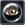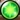## FANDOM

757 Pages

Template documentation follows
Note: the template above may sometimes be partially or fully invisible.
Visit Template:Orb/doc to edit this documentation. (How does this work?)

## Description

The Orb template is used to handle three types of item pages:

• Orbs
• Cores
• Shards

This template makes use of the Location template to display locations as links. The orb template will also make use of the Addcats to add several categories to the page and link to other pages.

In general when creating new pages, Orbs will make use of the following fields

• rarity
• stat
• bonus
• required level
• sell
• item
• quantity
• forge

If the orb is created in one step with just shards or gathered directly:

• chapter
• stage

Core pages make use of the following fields:

• rarity
• required level
• sell
• item
• quantity
• forge
• chapter
• stage

And finally Shard pages

• rarity
• required level
• sell
• chapter
• stage

When creating pages the item field is entered as a category, if the category page is new it should be added to the hidden category field by creating the category page and adding the line __HIDDENCAT__

## Syntax

```{{Orb
|altimage =
|rarity   =
|stat1    =
|bonus1   =
|stat2    =
|bonus2   =
|stat3    =
|bonus3   =
|stat4    =
|bonus4   =
|stat5    =
|bonus5   =
|stat6    =
|bonus6   =
|stat7    =
|bonus7   =
|stat8    =
|bonus8   =
|required level =
|sell      =
|item1     =
|quantity1 =
|item2     =
|quantity2 =
|item3     =
|quantity3 =
|item4     =
|quantity4 =
|forge     =
|chapter1  =
|stage1    =
|chapter2  =
|stage2    =
|chapter3  =
|stage3    =
|chapter4  =
|stage4    =
}}
```

## Example

```{{Orb
|altimage = Eye Orb icon.png
|stat1  =  SPD
|bonus1 =  +1%
|stat2  =  EVA
|bonus2 =  +1%
|required level = 1
|sell   = 400
|item1 = Power Orb
|quantity1 = 10
|forge  = 800
}}
```Orbs

### Orb### Stats

 SPD +1% EVA +1%
Requires Hero Level: 1
Sell:
400### Obtain

Forge:
800```{{Orb
|stat1  =  SPD|bonus1 =  1
|stat2  =  EVA|bonus2 =  1
|stat3  =  ATK|bonus3 =  +5
|stat4  =  DEF|bonus4 =  5%
|chapter1 = 1|stage1   = 1
|required level = 1
|sell   = 400
}}
```Orbs

### Orb### Stats

 SPD 1 EVA 1 ATK +5 DEF 5%
Requires Hero Level: 1
Sell:
400### Obtain

Component Location:
Location:

```{{Orb
|stat1  =  SPD|bonus1 =  1
|stat2  =  EVA|bonus2 =  1
|stat3  =  ATK|bonus3 =  +5
|stat4  =  DEF|bonus4 =  +5
|stat5  =  MATK|bonus5 =  5%
|stat6  =  MDEF|bonus6 =  5%
|required level = 1
|sell   = 400
}}
```Orbs

### Orb### Stats

 SPD 1 EVA 1 ATK +5 DEF +5 MATK 5% MDEF 5%
Requires Hero Level: 1
Sell:
400### Obtain

```{{Orb
|stat1  =  SPD|bonus1 =  +1%
|stat2  =  EVA|bonus2 =  +1%
|stat3  =  ATK|bonus3 =  +5%
|stat4  =  DEF|bonus4 =  +5%
|stat5  =  MATK|bonus5 =  +5%
|stat6  =  MDEF|bonus6 =  +5%
|stat7  =  MATK|bonus7 =  +5%
|stat8  =  MDEF|bonus8 =  +5%
|required level = 1
|sell   = 400
|item1 = Power Orb|quantity1 = 1
|item2 = Magic Orb|quantity2 = 2
|item3 = Agility Orb|quantity3 = 4
|item4 = Shooting Orb|quantity4 = 8
|forge  = 800
|chapter1 = 1|stage1   = 2
|chapter2 = 2|stage2   = 3
|chapter3 = 3|stage3   = 4
}}
```Orbs

### Orb### Stats

 SPD +1% EVA +1% ATK +5% DEF +5% MATK +5% MDEF +5% MATK +5% MDEF +5%
Requires Hero Level: 1
Sell:
400### Obtain